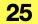Describes the Structural Analytical Model Analytical Point Load settings.

```    typedef enum {
API_Force,
API_Moment
```
```    typedef struct {
API_AnalyticalCoordSysDirection        coordinateSystemType;
double                                 xValue;
double                                 yValue;
double                                 zValue;
```

#### Members

loadCaseIdThe guid of the connected Load Case.
loadForceTypeThe force type of the Analytical Point Load. The point force can be defined in three main directions (global or local coordinate system). A node of the structure may be subject to a moment load. The load is defined by the direction and size of the moment.
coordinateSystemTypeThe coordinate system type of the Analytical Point Load.
xValueThe force/moment value in X direction of the Analytical Point Load
yValueThe force/moment value in Y direction of the Analytical Point Load
zValueThe force/moment value in Z direction of the Analytical Point Load

#### Requirements

Version: API 25 or later